# Week 1: A First Plot

Tutorial
Get with the Plot!
Data Visualization
R
Create your first plot and learn about the elements that make up ggplot2.
Author
Published

October 9, 2018

This is the first of a series of posts on how to use `ggplot2` to visualise data in R.

We begin by loading the `tidyverse` package which contains `ggplot2` as well as a host of other useful packages. If you haven’t yet, you first need to install the `tidyverse` package by running `install.packages("tidyverse")`.

``library(tidyverse)``

Next, we need some data. For this post, we download a dataset from my data visualisation lecture.1

``d1 <- read_rds("https://github.com/nilsreimer/data-visualisation-workshop/raw/master/materials/d1.rds")``

Before plotting anything, we should get to know the data. We look at the first five rows of the dataset.

``print(d1, n = 5)``
``````# A tibble: 161 × 4
V1    V2 V3           V4
<dbl> <int> <chr>        <chr>
1   3.7    59 Experimental Group 1
2   3.4    45 Control      Group 2
3   3.5    49 Control      Group 1
4   2.8    48 Experimental Group 2
5   4.2    90 Experimental Group 2
# ℹ 156 more rows``````

From this, we learn that the dataset contains 161 observations of 4 variables—two numeric variables and two character variables. `V1` and `V2` could be, for example, responses to survey items, while `V3` and `V4` seem like grouping variables. We start by plotting the two numeric variables (`V1`, `V2`) against each other. We might do this if we were interested in the relationship between the two variables.

In Excel or SPSS, we would do this by selecting the scatterplot from a list of various visualisations. Point-and-click programs tend to rely on a typology of visualisations, limiting you to one kind of visualisation per plot. `ggplot2` is different in that it uses a grammar of graphics, allowing you to build your plot from various elements described by its grammar.

We begin by setting up our plot using the `ggplot()` function.

``ggplot(data = d1, mapping = aes(x = V1, y = V2))``

The `ggplot()` function has two arguments. `data` defines which dataset to use for the plot; this allows us to refer to `V1` and `V2` without repeating from which dataset they come. `mapping` defines which variables are mapped onto which aesthetics. Aesthetics are the visual properties of the elements that make up the plot. For example, `aes(x = V1, y = V2)` means that the `x` and `y` aesthetics of all elements will be mapped onto the variables `V1` and `V2`, respectively. I promise this will all make sense once we get to some concrete examples.

We don’t usually have to spell out `data` and `mapping`.

``ggplot(d1, aes(x = V1, y = V2))``Running this function creates an empty grid with the two axes we have mapped onto the `V1` and `V2` variables. `ggplot2` implements a layered grammar of graphics. The `ggplot()` function creates a base layer with some useful defaults—for example, that the x- and y-axis roughly correspond to the range of the data. We can add other elements on top of that layer using the `+` operator.

``````ggplot(d1, aes(x = V1, y = V2)) +
geom_point()```````geom_point()` adds a layer of points on top of the base layer. Geoms are the geometric shapes that make up `ggplot2` visualisations. Each is called with a function that begins with `geom_*` and ends with the name of the geom (e.g., `point` or `line`). Each geom has a number of aesthetics that define its visual properties. In this case, `geom_point()` inherits the `x` and `y` aesthetics from the `ggplot()` function. We can add other aesthetics.

``````ggplot(d1, aes(x = V1, y = V2)) +
geom_point(size = 2)``````Unsurprisingly, the `size` aesthetic influences the size of the points in this layer. Note that we have specified the `size` argument outside the `aes()` function. Let’s add another aesthetic inside the `aes()` function to illustrate the difference.

``````ggplot(d1, aes(x = V1, y = V2)) +
geom_point(aes(shape = V3), size = 2)```````aes(shape = V3)` maps the `shape` aesthetic onto the categorical `V3` variable, and adds the corresponding legend to the plot. Had we instead included `shape = "triangle"` outside the `aes()` function, all points would become triangles. To find all aesthetics that apply to the `geom_point()` function, call the `help()` function by running `?geom_point`. You can find a useful list of all aesthetic specifications here.

At this point, we could add other `geom_*` layers by using the `+` operator—but we’ll leave this for future posts. Instead, we add another kind of element to the plot.

``````ggplot(d1, aes(x = V1, y = V2)) +
geom_point(aes(shape = V3), size = 2) +
scale_x_continuous(limits = c(1, 7), breaks = 1:7) +
scale_y_continuous(limits = c(0, 100))``````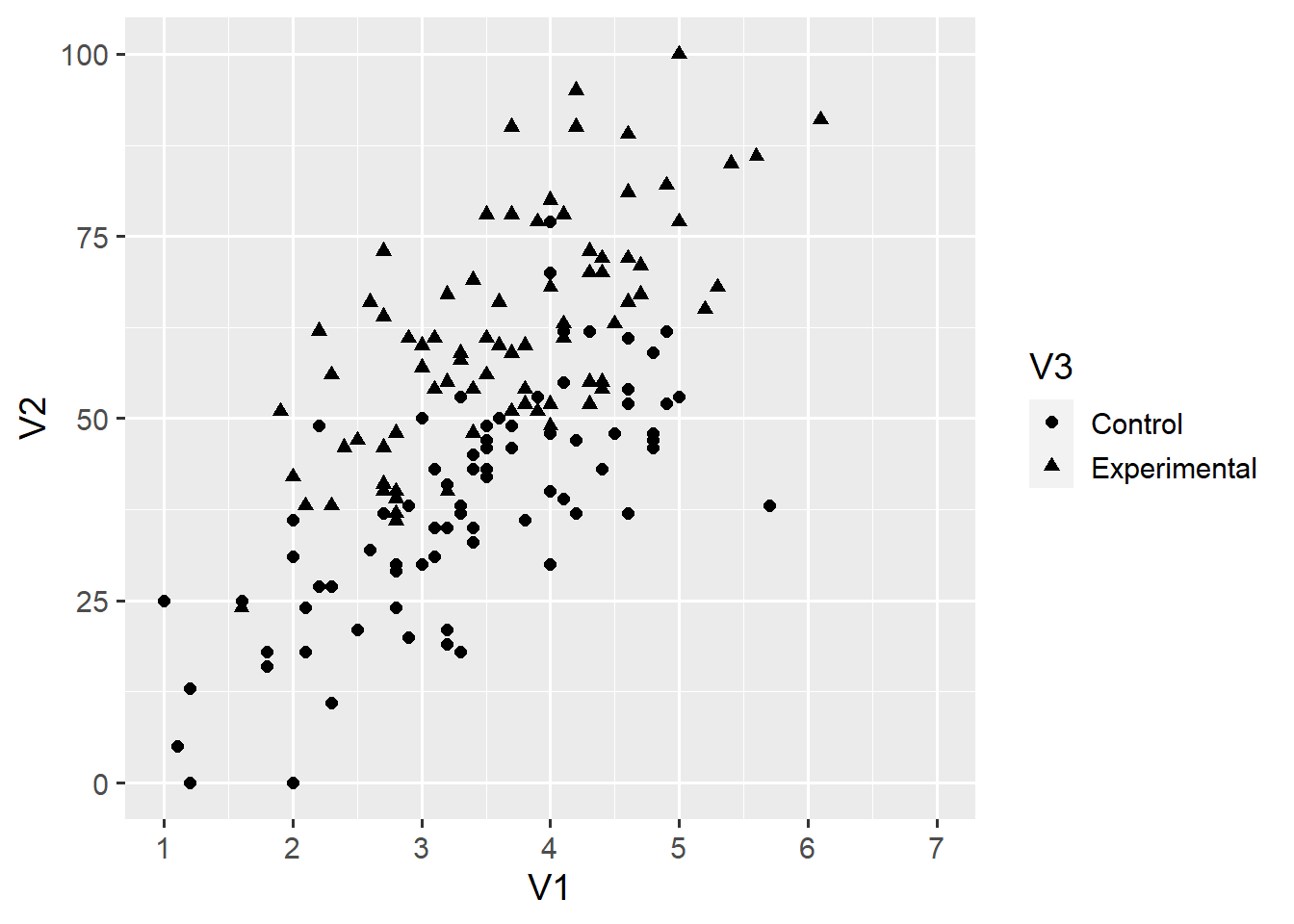Each aesthetic has a corresponding scale that takes the form `scale_*`. We don’t have to spell out each scale as long as we are happy with the default. Above, we have called the `scale_x_continuous()` function to change the scale for the x-axis. The `limits = c(1, 7)` argument forces `ggplot2` to have an x-axis ranging from `1` to `7`. The `breaks = 1:7` argument labels all integers from `1` to `7` on the x-axis. We can add another scale for the `shape` aesthetic.

``````ggplot(d1, aes(x = V1, y = V2)) +
geom_point(aes(shape = V3), size = 2) +
scale_x_continuous(limits = c(1, 7), breaks = 1:7) +
scale_y_continuous(limits = c(0, 100)) +
scale_shape_discrete(solid = FALSE)``````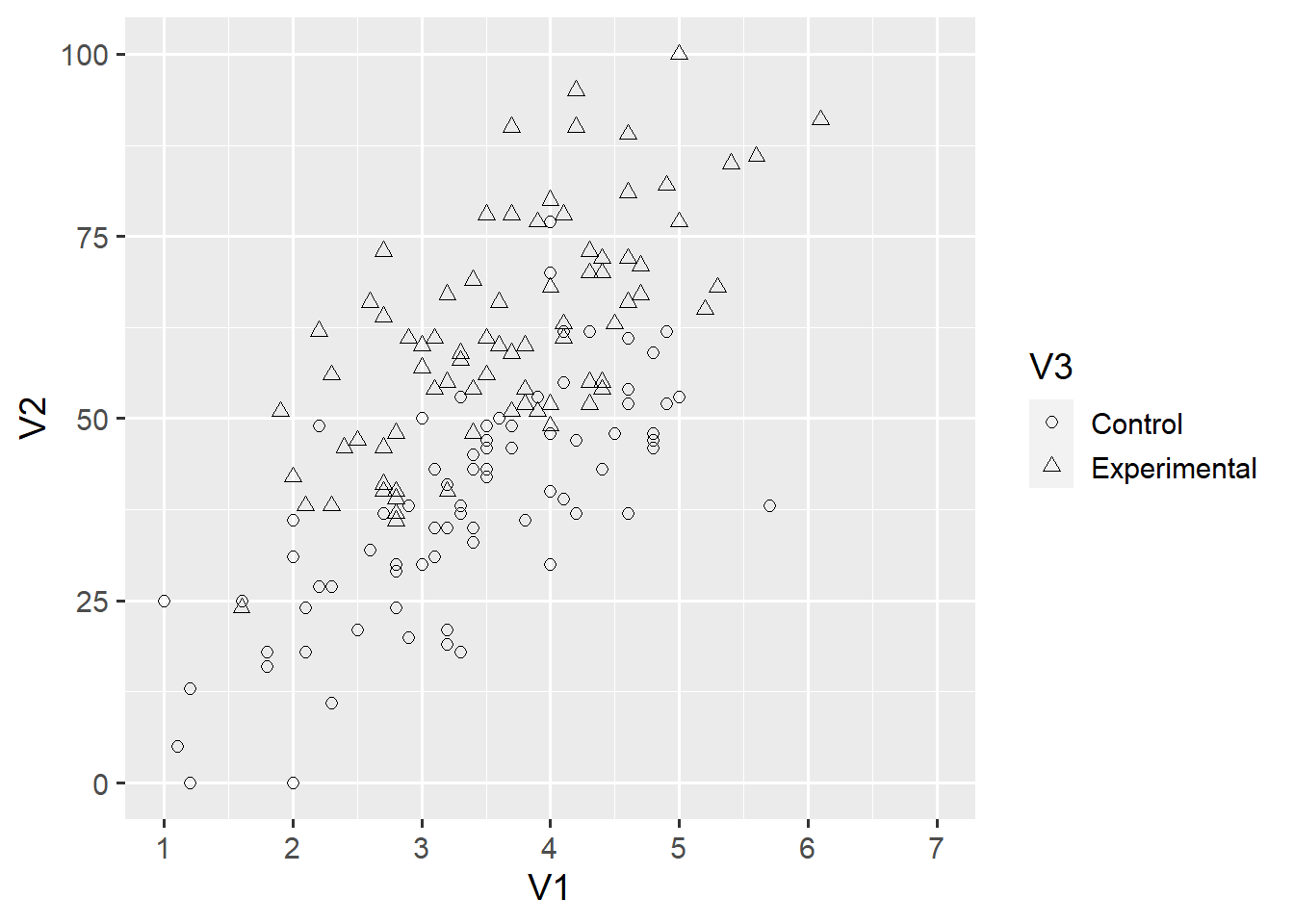Again, you can use the `help()` function to find out what you can change about each scale. Another element of the `ggplot2` grammar are coordinate systems. By default, `ggplot()` assumes a Cartesian coordinate system (`coord_cartesian`). Here, we use `coord_fixed()` to define a Cartesian coordinate system with a fixed axis ratio.

``````ggplot(d1, aes(x = V1, y = V2)) +
geom_point(aes(shape = V3), size = 2) +
scale_x_continuous(limits = c(1, 7), breaks = 1:7) +
scale_y_continuous(limits = c(0, 100)) +
coord_fixed(ratio = 6/100)``````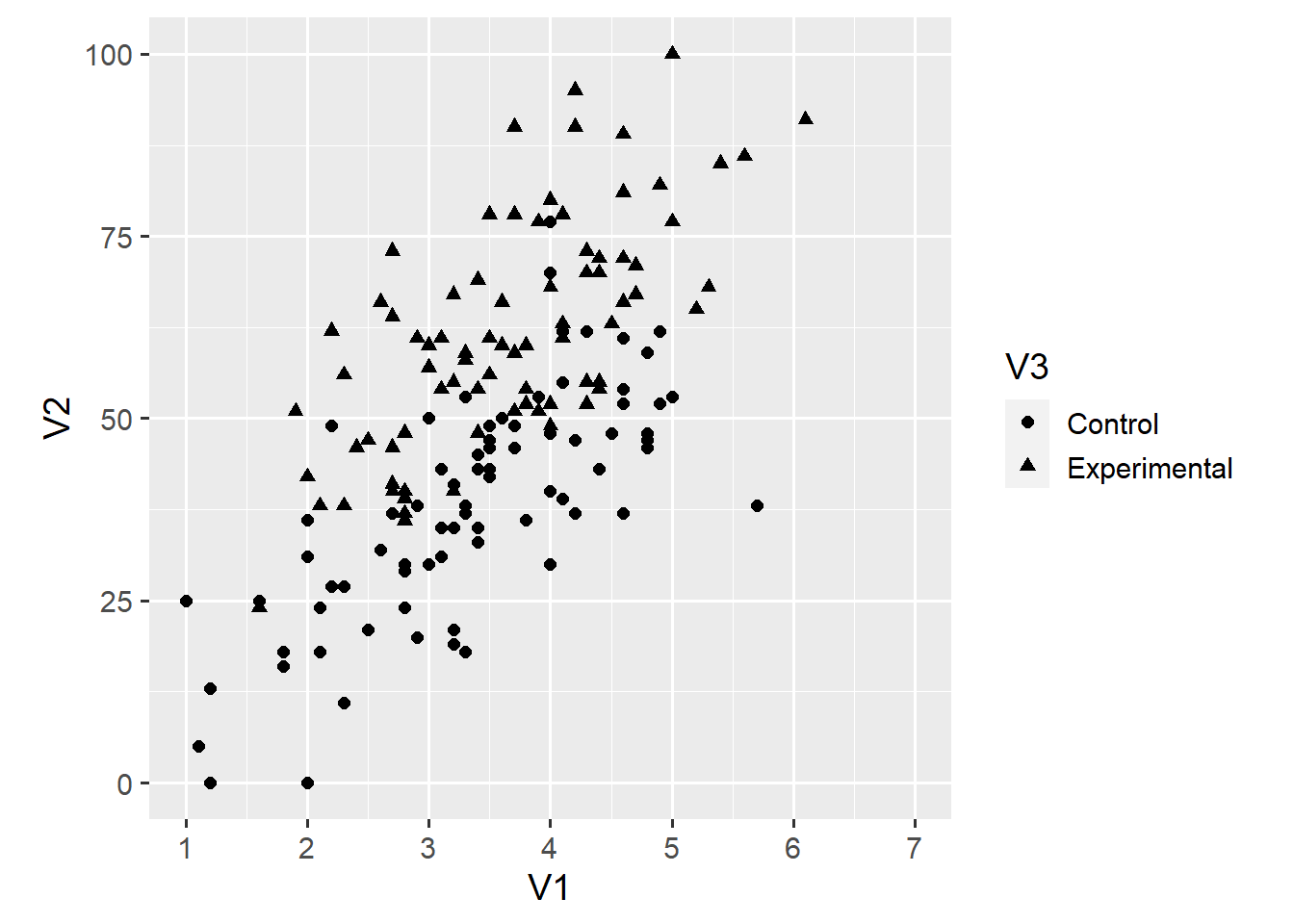In this case, the `ratio = 6/100` argument creates a square plot. Coordinate systems can be very useful as we’ll discuss in a future post. Thus far, we have used variables `V1`, `V2` and `V3` in our visualisation. `ggplot2` is flexible enough to also include the remaining variable. For example, we can use the `colour` aesthetic to map each point’s colour onto the `V4` variable.

``````ggplot(d1, aes(x = V1, y = V2)) +
geom_point(aes(shape = V3, colour = V4), size = 2) +
scale_x_continuous(limits = c(1, 7), breaks = 1:7) +
scale_y_continuous(limits = c(0, 100)) +
coord_fixed(ratio = 6/100)``````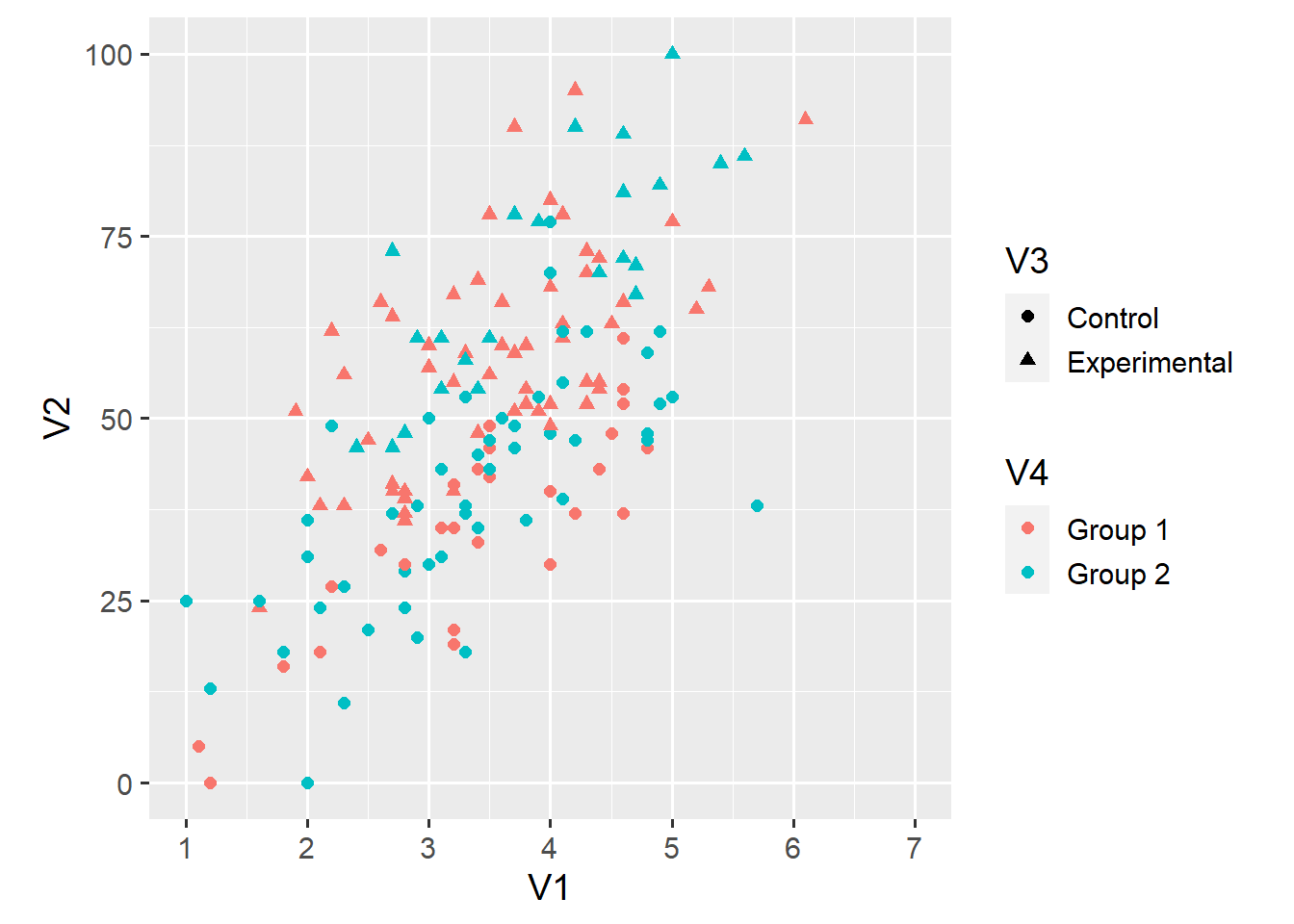This argument also adds a second legend to the plot. This change shows two things—first, that you can do a lot with `ggplot2` and, second, that you probably shouldn’t. Looking at this plot, I find it hard to say whether the relationship between `V1` and `V2` depends either on the condition (`V3`) or the group (`V3`) of an observation.

This brings us to the last element I want to introduce. `ggplot2` makes it very easy to create small multiples of a plot. Here, we use the `facet_grid()` function to create separate plots for each value of `V4`.

``````ggplot(d1, aes(x = V1, y = V2)) +
geom_point(aes(shape = V3, colour = V4), size = 2) +
scale_x_continuous(limits = c(1, 7), breaks = 1:7) +
scale_y_continuous(limits = c(0, 100)) +
coord_fixed(ratio = 6/100) +
facet_grid(. ~ V4)``````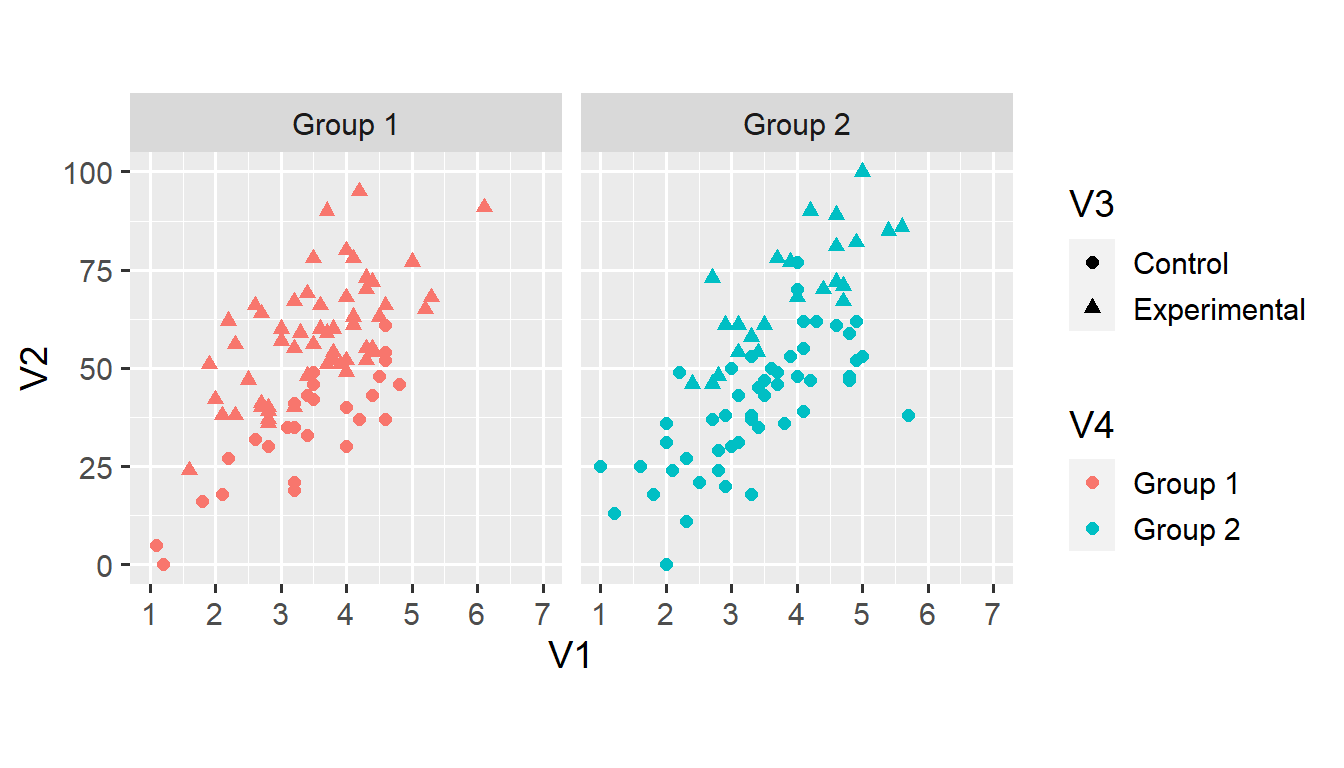We’ll cover faceting in more detail later in this series. For now, note that faceting makes it much easier to examine the relationship between `V1` and `V2` across the two categorical variables. However, the `colour` doesn’t seem particularly useful in the new plot. Instead, we can map both `shape` and `colour` onto variable `V3`.

``````ggplot(d1, aes(x = V1, y = V2)) +
geom_point(aes(shape = V3, colour = V3), size = 2) +
scale_x_continuous(limits = c(1, 7), breaks = 1:7) +
scale_y_continuous(limits = c(0, 100)) +
coord_fixed(ratio = 6/100) +
facet_grid(. ~ V4)``````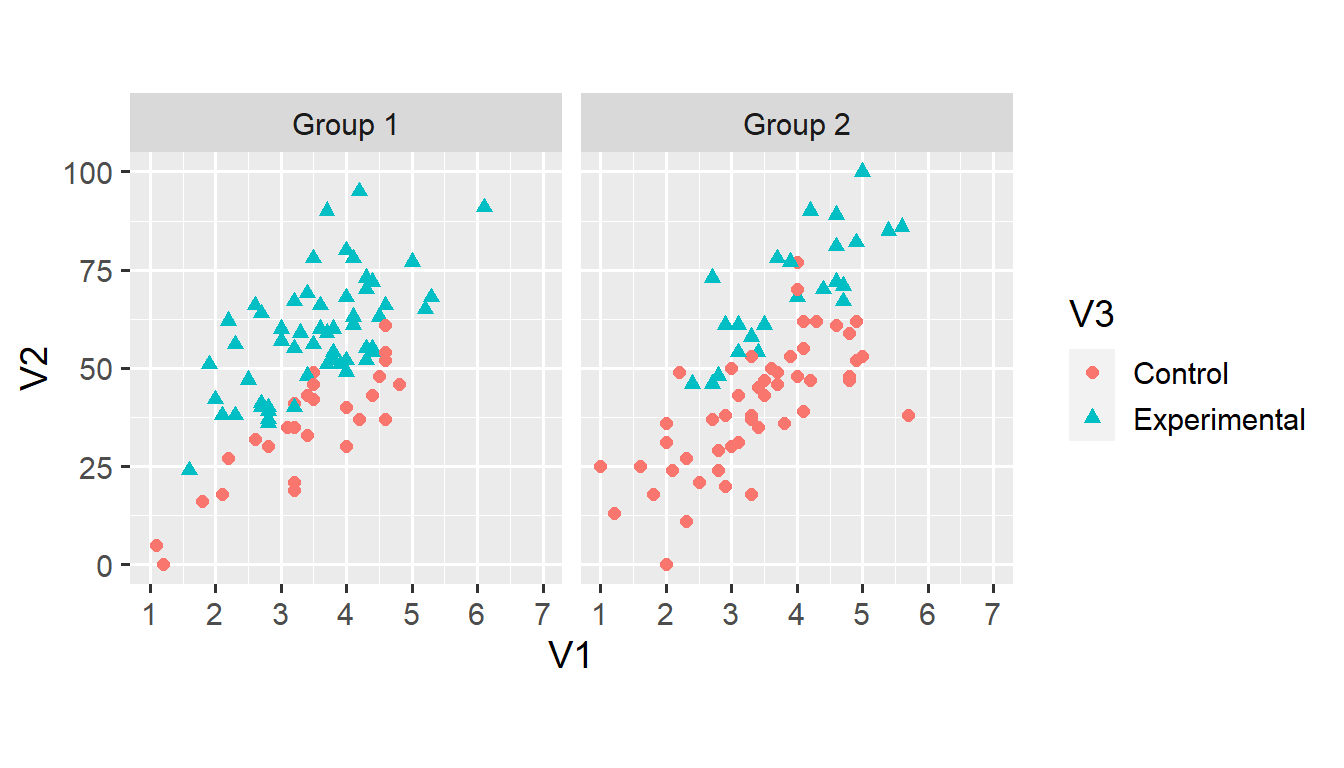Note that the legend now reflects both aesthetics. Mapping two aesthetics onto the same variable sometimes makes for a better plot. In this case, the `shape` aesthetic makes the plot more accessible for people with colour vision deficiencies.

And that’s it for this post. You now understand the basic elements of `ggplot2` and are well on your way towards building more complex data visualisations. If you have a question—or found a mistake—please comment on Twitter or send me an email.

There are other elements that we’ll cover in future posts—for example, `stat_*` layers or the `theme()` function. Next week, we’ll continue working with this dataset. I’ll write some more about faceting, and introduce some new geoms and aesthetics.

## Footnotes

1. If this command doesn’t work, download the file from here and run `d1 <- read_rds(".../d1.rds")` after replacing `...` with the path to the folder into which you’ve downloaded the file.↩︎